# Array Fun 2 

Complete all the methods below. When you are done, copy and paste the testmethods.v4  into your class and run it to see if you have any obvious errors.

int sumEveryN(int[] nums, int n)

Description: This method returns the sum of every `n` elements of `num`s..

 Method Call return value/output sumEveryN( {1 , 2 , 3 , 4 }, 2 ) 4( ie 1 +3) sumEveryN( {13 , 42, 15, 33 , 44 , 16 , 52} ,3) 98 ( ie 13 + 33+ 52)

String[] doubleArr(String[] strs)

Description: This method returns a new version of `strs` in which each element now appears twice. This can be done with a for-each loop, which I believe is easier and more intuitive.

 Method Call return value/output doubleArr( {“a”,”b”,”c”} ) {“a”,”a”,”b”,”b”, “c” , “c”} doubleArr( {“math”,”ware”,”house”,”.com” }) {“math”,”math”,”ware”,”ware”,”house”,”house”,”.com”, “.com”}

int  indexOf5(int[] nums )

Description:This returns the index of the first occurrence element 5  or -1 if 5 does not appear anywhere in the array.

 Method Call return value/output indexOf5( { 2 , 3 , 5 , 4 } ) 2 indexOf5( { 2 , 3 , 5 , 4, 5  } ) 2 indexOf5( { 2 , 3 ,7  , 4, 3,   } ) -1

More Sample calls and return vals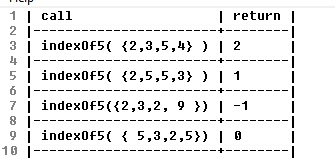int indexOf(int[] nums, int num)

Description: This method returns the index value of the first appearance of `num` or -1 if `num` is not an element of `nums` .

 Method Call return value/output indexOf( {6,4 ,7,3, 4 }, 4) 1 indexOf( {6,4 7 ,3,2,7}, 7) 2 indexOf( {6,4 ,2,3}, 22) -1

int[] randos(int start, int end, int howMany)

Description:  This method returns an array of random numbers between [start,end] . Note make sure that each element in the new array attempts to make a new random int.

More example calls and returns :

double meanBetween(int[] nums, int min, int max)

Description:  This method returns the mean of nums ; however, this method only counts values within the range (min,max) as shown in the examples below:

int secondSmallest(int[] nums )

Description: This method returns the element of `nums` with the second smallest value.

Note: You may not modify the input array. For instance, you may not put nums in order,  which would be bad because you were not asked to modify the array.

@precondition: nums.length >= 2

Note: You will lose credit if you use a constant to represent the smallest or second smallest number. See pseudocode

 Method Call return value/output secondSmallest( { 2 , 18 , 22, 4 , 6 } ) 4 secondSmallest( { 3 , 7 , 15 , 1 ,101} ) 3

The question below is extra credit .
** int[] add(int[] num1, int[] num2, int base) throws ArithmeticException
@precondition `1 < base < 11`
@precondition `num1.length = 5 and num2.length = 5`
@postcondition: the returned array has 5 elements representing the sum or an ArithmeticException is thrown

Description: This method attempts to replicate addition. Consider both num1 and num2 represent the five digits of a number. Each element in the array stores one of the digits. For instance, the number 143 would be represented as {0,0,1,4,3 }. Numbers can be in any base between 2 and 10 inclusive , and the number 101102 in binary would appear in an arrays as : {1, 0, 1 , 1, 0 } . Let’s assume the numbers are positive. You should return an array representing the digits of the sum of num1 and num2.  You may not do any math besides addition. You may not use any external libraries for any math or base conversions.   If the number of digits in the sum exceeds the maximum number of digits (5) , you should throw an arithmetic exception as shown in the code below:

Note: You may not use any kind of helper or utility methods that are built into pre-defined Java classes for converting numbers between bases. Only use techniques taught in this class.

 Method Call return value/output add( {0,0,0 ,4,2},{0,0,0,5,1}, 10) {0, 0, 0, 9, 3} ie (42 + 51 = 93) add( {0, 0, 0 ,7,2},{0,0,0,5,1}, 10) {0, 0 , 1 , 2, 3} ie (72 + 51 = 123) add( {0, 0 , 0 , 1 , 1}, { 0 , 0 , 0 , 1 }, 2) {0, 0 , 1 , 0 , 0} ie ( 112 + 12= 1002 )

Old versions:

Array Fun 1

Array F un 2 (v 1)

Array Fun 3 (resizing included)

# String 1 and Array 1 Exercises

Exercises for String 1 and Array 1 (no loops)

When you are done, you can copy and past the score() method below into your class. It will test some (but, by no means all) of the things that your code should do

Create a class called StrArr1 and add each method below into it.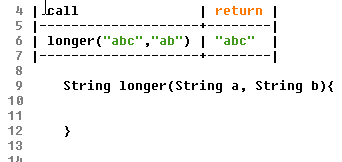For the absVals()  method below use the Math.abs() Java method.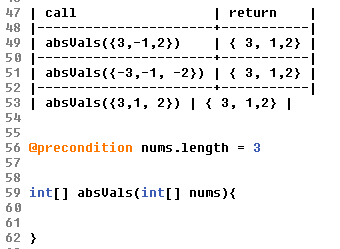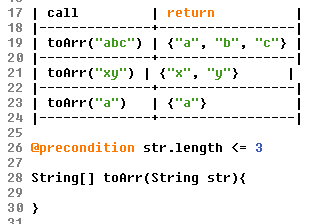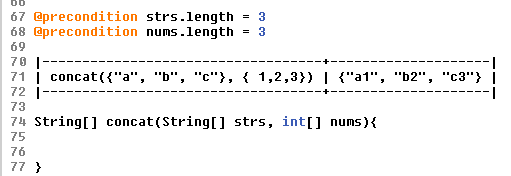For the  randos1to10()   method below use the  Math.random()  Java method. Create a new array to store random integers. Each integer should be [1,10]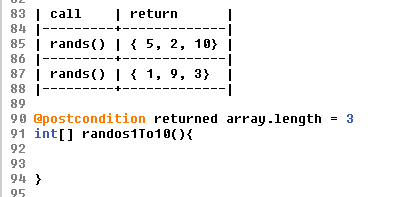# Class Roster Array Version [Java] Assignment

Class Roster: This class will implement the functionality of all roster for school. It will , in essence, manage an Array of Student objects. Each roster will store a default of 10 Students

• “global” Instance Variables
• private Student myStudents   : an array storing Student Objects
• private String className  (a name to represent a given roster like “Ap CS”)
• public int period //during which period of the day does this roster meet
• a default constructor should initialize the Array to store 20 strings
a single parameter constructor that takes an Array <String>
Both constructors should initialize the instance variables
• Methods
• Accessors
• private int indexOf(Student st) //@returns index of Student St or -1
• public boolean containsStudent(Student studentName )/ /@returns true if studentName is in roster .
• public boolean equals(ClassRoster other) //@ returns whether or not rosters are equal. Rosters are equal if identical students are in identical order in the lists
• Mutators:

public void addStudent(String studentName, int age) ;// adds student name and age to end of roster

. Hint: Make user of the containsStudent() method
public boolean removeStudent(int ssnId ) // removes student from roster based on id. Make sure that you maintain the integrity of the parallel ArrayLists.

public boolean removeStudent(String name) //removes student based on name. Make sure that you maintain the integrity of the parallel ArrayLists.

public  boolean removeStudent( Student st) //removes student st. Make sure that you maintain the integrity of the parallel ArrayLists.

# Student Class

A school district decided to write a program to help keep track of various parts of the school.

It has been decided to store relevant information about Students in a Student class. Each Student will have an array of Strings to store the names of his or her teachers.

private instance variables

Part 1

* Construct the Java statement to declare the array called

teachersNames to store the String names of the student’s teachers

Part 2

• write a default constructor
• construct a method called  addTeacher( String name) that adds String name to the  teachersNames  array
• to do this let’s create a private method  private String[] addStr( String[] input, String val) that adds value to the end of the array and returns it. [redact]Solution[/redact]
• construct hasTeacher(String name)  that returns true if name is in the teachersName array
• construct a method removeTeacher(String name) that removes the   String name from the  teachersNames  array.
• construct a method the line of code needed in the setGpa(double to) method of the Student Class.
• construct a method boolean setTeacher (String name, int index) .   This method should return true if index is valid in the array and false if  index >= teachersName.length

Next: The runner class

# Java AssignmentCreate a Class called ArrayFun2  that has the following methods Write the body for the methods described below.

Description: This method creates an array that is filled with all of the digits of `nums`.

 Method Call return value/output digitsToArray( 523 ) {3, 2, 5} digitsToArray(1267 ) { 7 , 6 , 2,  1 }

public boolean allFactorsOfSum(int[] nums)

Description: This method returns true if each element of`nums` is a factor of the sum of `nums`.

 Method Call return value/output allFactorsOfSum( {6,1,2,3} ) true (because all of the elements are factors of the sum which is12) allFactorsOfSum( 1,4,7) false (because 7 is not a factor of the sum of this array which is 12)

public String[] doubleArr(String[] strs)

Description: This method returns a new version of `strs` with each element appearing twice.

 Method Call return value/output doubleArr( {“a”,”b”,”c”} ) {“a”,”a”,”b”,”b”, “c” , “c”} doubleArr( {“math”,”ware”,”house”,”.com” }) {“math”,”math”,”ware”,”ware”,”house”,”house”,”.com”, “.com”}

public boolean isThere(int[] nums, int num)

Description: This method returns true if `num `is an element `nums`.

 Method Call return value/output isThere( {6,1,2,3}, 6) true isThere( {6,1,2,3}, 5 ) false

public int indexOf(int[] nums, int num)

Description: This method returns the index value of the first appearance of `num` or -1 if `num` is not an element of `nums` .

 Method Call return value/output indexOf( {6,4 ,7,3, 4 }, 4) 1 indexOf( {6,4 7 ,3,2,7}, 7) 2 indexOf( {6,4 ,2,3}, 22) -1

public int lastIndexOf(int[] nums, int num)

Description: This method returns the index value of the last appearance of `num` or -1 if `num` is not an element of `nums` .

 Method Call return value/output lastIndexOf( {6, 4 ,7 ,3, 11, ,4}, 4) 5 lastIndexOf( {7, 6,4 , 7 ,3}, 7) 3 lastIndexOf( {6,4 ,2,3}, 22) -1

public boolean isIncreasing(double[] nums)

Description: This method returns true if each element in nums is greater than the element to its left.

 Method Call return value/output isIncreasing( {1,2,3,4 }) true isIncreasing( {1,0 ,3,4 }) false isIncreasing( {1,1, 2,3,4 }) false

public int largestSpan(int[] nums)

Description: This method returns the largest span of consecutive increasing numbers

 Method Call return value/output largestSpan( {4, 3, 1, 2, 3 , 1} ) 3 largestSpan( {4  , 3,  1 , 12 , 31 , 44 , 52 , 1} ) 5 largestSpan( {9, 7, 8 , 12,4, 3, 0, 4 , 1) 3 largestSpan( {1, 0, 4, 5 , 3,  2, 8 , 9, 10, 12, 4, 3,  1 }) 5

** int[] invert(int[] nums)

Description: This method ‘inverts’ an array by spliting the array in halves and ‘inverting’ each half. See sample calls to understand.

 Method Call return value/output invert( {5 , 21, 5, 13,4 }) {4, 13, 5, 21, 5  } invert( { 1, 2, 3, 4, 5,6  }) { 6, 5, 4, 3, 2, 1}

** int[] shiftByN(int[] nums, int delta)

Description: This method returns the array with all digits shifted by `delta` . Any digits that are circulated off the end of the array should be returned to the other side. Note: delta could be positive or negative. Please keep in mind that Math.abs(delta) > nums.length could be possible 😉

 Method Call return value/output shiftByN( {5 , 21, 13,4 } 1) { 4 , 5 , 21, 13 } shiftByN( {5 , 21, 13, 4 , 11} 2) { 4 , 11 , 5 , 21, 13} shiftByN( {5 , 21, 13, 4 } -1) { 21, 13, 4 , 5} shiftByN( {5 , 21, 13, 4 ,7 } -2) { 13, 4 ,7 , 5 , 21}
** int[] add(int[] num1, int[] num2, int base) throws ArithmeticException@precondition `1 < base < 11`
@precondition `num1.length = 5 and num2.length = 5`
@postcondition: the returned array has 5 elements representing the sum or an ArithmeticException is thrown

Description: This method attempts to replicate addition. Consider both num1 and num2 represent the five digits of a number. Each element in the array stores one of the digits. For instance, the number 143 would be represented as {0,0,1,4,3 }. Numbers can be in any base between 2 and 10 inclusive , and the number 101102 in binary would appear in an arrays as : {1, 0, 1 , 1, 0 } . Let’s assume the numbers are positive.You should return an array representing the digits of the sum of num1 and num2. If the number of digits in the sum exceeds the maximum number of digits (5) , you should throw an arithmetic exception as shown in the code below:

Note: You may not use any kind of helper or utility methods that are built into pre-defined Java classes for converting numbers between bases. Only use techniques taught in this class.

 Method Call return value/output add( {0,0,0 ,4,2},{0,0,0,5,1}, 10) {0, 0, 0, 9, 3} ie (42 + 51 = 93) add( {0, 0, 0 ,7,2},{0,0,0,5,1}, 10) {0, 0 , 1 , 2, 3} ie (72 + 51 = 123) add( {0, 0 , 0 , 1 , 1}, { 0 , 0 , 0 , 1 }, 2) {0, 0 , 1 , 0 , 0} ie ( 112 + 12= 1002 )